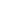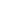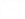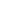Overview

Photonic Arithmetic Computing Engine

PACE (Photonic Arithmetic Computing Engine) is Lightelligence' s first fully integrated optical computing platform. The core of PACE is a 64x64 optical matrix multiplier in an integrated silicon photonic chip and a CMOS microelectronic chip, flipchip packaged together. In addition to its advanced 3D packaging, PACE's photonic chip contains over 12,000 discrete photonic devices and has a system clock of 1GHz.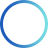64x64

oMAC core

150ps

oMAC Computing Delay
Request Documentation

Key Technology

oMAC – OPTICAL MULTIPLY ACCUMULATE

For each optical Matrix Multiplication (MATMUL), the input vector values are first extracted from the on-chip SRAM before being turned into analog values by the digital-analog converter. Next, the values are applied to their correspond...

For each optical Matrix Multiplication (MATMUL), the input vector values are first extracted from the on-chip SRAM before being turned into analog values by the digital-analog converter. Next, the values are applied to their corresponding optical modulators through micro bumps between the electronic and photonic chips to form the input optical vector. The input optical vector then propagates through the optical matrix to generate the output optical vector. The vector then hits an array of photodetectors, which turn optical intensity into electric current. The electrical signals then travel back to the electronic chip through micro bumps and pass through the transimpedance amplifier and the analog-digital converter before returning to the digital domain.

Click to expand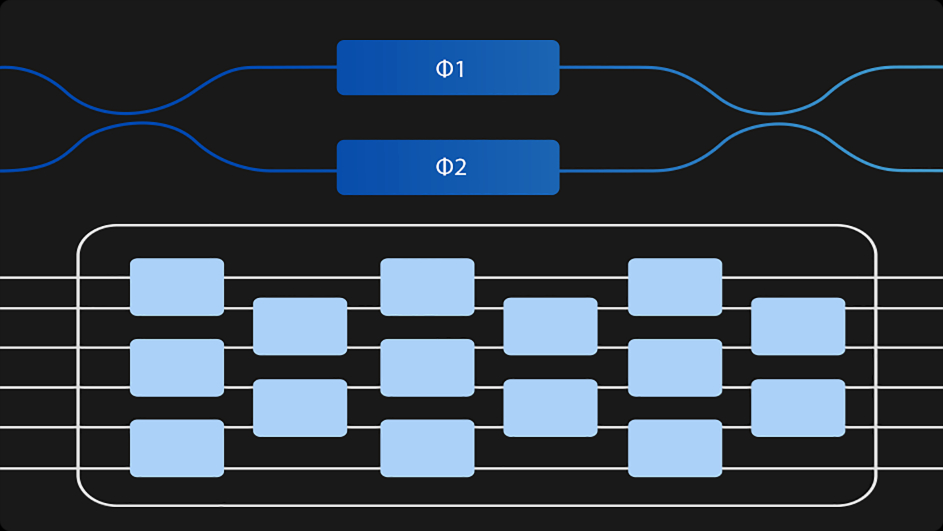Approach

Based on a silicon photonics platform compatible with CMOS processes, optical-electrical co-design, and advanced packaging solutions

Novel design using high speed, configurable, compact modulators

Unique computing architecture: coherent and incoherent architectures

Based on MZI structure

Hardware-algorithm co-optimizationHighlightsStrong parallel abilityUltra-low
latencyEnergy efficiency
comparable to EICLow-cost and low process requirements

Demonstration

PACE is designed to solve the lsing problem for two cases, with N=63 and N=64 spins. The interaction between every two spins is encoded by the NxN-size matrix H, the adjacency matrix of the graph of the Ising model. The state of all the spins is described by the N-size binary vector  S, called the state vector.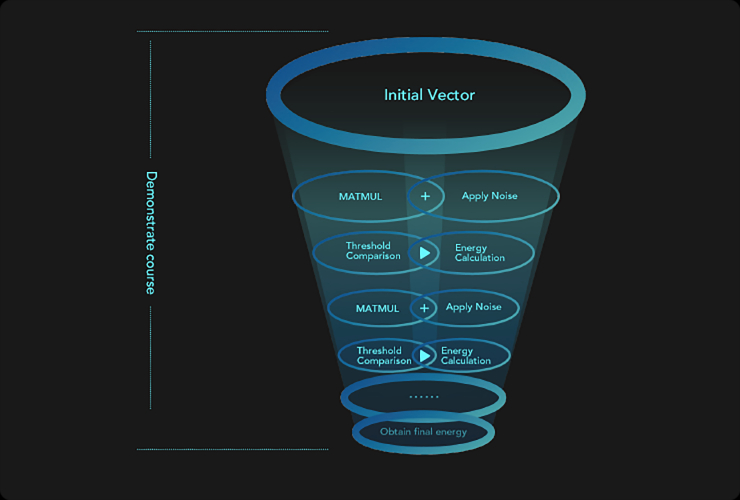The following are the steps for the algorithm

Initialize the state vector S randomly.

Loop 5,000 times and in each loop:

• Convert the state vector S from digital to optical

• Perform a noisy multiplication of the matrix H and the vector S on the photonic chip

• Convert the product HS from optical to analogic domains

• Apply a threshold getting an updated state vector

• Convert the updated state vector from analogic to digital domains

• Digitally calculate an energy of the updated state vector

Obtain the final state vector as that having the smallest energy by the end of 5,000 iterationsUsage Scenario

LIGHT UP THE FUTURE OF CHIPS

Bioinformatics

Provide efficient computing power for bioinformatics researchBioinformatics

Provide efficient computing power for bioinformatics research

Route Planning

Accelerate various route planning algorithms, which can be appliedRoute Planning

Accelerate various route planning algorithms, which can be applied

Material Research & Development

Accelerate simulation calculations in new material research for processing and manufacturing industriesMaterial Research & Development

Accelerate simulation calculations in new material research for processing and manufacturing industries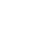Technical documentation